Science Research
Volume 4, Issue 6, December 2016, Pages: 157-168

Online Adaptive Continuous Wavelet Transform and Fuzzy Logic Based High Precision Fault Detection of Broken Rotor Bars for IM

Ali Saghafinia1, S. Hr. Kaboli2

1Department of Electrical Engineering, Majlesi Branch, Islamic Azad University, Majlesi, Iran

2UM Power Energy Dedicated Advanced Centre (UMPEDAC), Wisma R&D, University of Malaya (UM), Jalan Pantai Baharu, Kuala Lumpur, Malaysia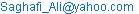(A. Saghafinia)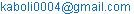(S. Hr. Kaboli)

Ali Saghafinia, S. Hr. Kaboli. Online Adaptive Continuous Wavelet Transform and Fuzzy Logic Based High Precision Fault Detection of Broken Rotor Bars for IM. Science Research. Vol. 4, No. 6, 2016, pp. 157-168. doi: 10.11648/j.sr.20160406.13

Received: November 24, 2016; Accepted: January 16, 2017; Published: February 7, 2017

Abstract: This paper presents an intelligent fault detection based on adaptive continuous wavelet transform of broken rotor bars for Induction Motor (IM). Broken rotor bars, bearing decay, eccentricity, as motor faults appears as different frequencies in the stator current signals. The stator current and speed signals at deferent operation conditions obtained from the winding function are analysed through the adaptive continuous wavelet transform (CWT) to detect the amplitudes and frequency components corresponding to different broken bar fault and load conditions. The adaptive coefficients of CWT based on the harmonics amplitude, are applied to train a fuzzy logic controller (FLC) in simulation. Then, detection of the fault condition are done based on the adaptive CWT and trained FLC in both simulation and real-time. The experimental results are confirmed the simulation results and show the effectiveness of the proposed method to detect the motor fault conditions accurately.

Keywords: Adaptive Continuous Wavelet Transform, Fault Detection, Squirrel Cage Induction Motor, Fuzzy Logic Controller, Broken Rotor Bars

Contents

1. Introduction

Over the years, Induction motors (IM’s) have some advantages features such as low cost, simple and robust construction and almost maintenance free. That is why, they are popular industry . IMs suffer from different broken bar fault conditions due to the motor work continuously. Diagnosis of broken bars for IM has been investigated for a long time. Traditionally, fast Fourier transform (FFT) method is applicable to detect fault detection purpose, widely [2-3]. However, in the case of load variation and speed changes, FFT of the rotor fault detection does not satisfy the appropriate accuracy, as the slip frequency varies with these variations [4,5]. In addition, fault detection with FFT it is not able to evaluate the frequency detection on-line during transient state. Some works are focused on the broken rotor bar detection of IM using discrete wavelet transform (DWT)  and wavelet package decomposition (WPD) . A few works has been reported on continuous wavelet transform (CWT) for fault detection of IM . However, in  authors presented a single phase stator current signal, which is not enough to detect the motor faults, accurately and the CWT was not adaptive in nature.

Over the past two decades, artificial intelligent algorithms have been utilized for motor drives, fault detection, process control [8-17]. In order to fuzzy fault detection considering the advantage of CWT, this paper presents an accurate fault detection through adaptive CWT and fuzzy logic controller (FLC). The proposed fault detection method is checked in both simulation and experiment at different operation conditions. The results show the effectiveness of the online broken rotor bars fault detection of IM. The superiority of this method to the conventional FFT method is also verified by the results.

2. Control Techniques

The control techniques to train the FLC and detect the fault for IM with broken rotor bars are shown in Figure 1 and Figure 2, respectively. The IM is first modelled by using the modified winding function method (WFM), under various load and relative failure conditions with considering the saturation effects . The CWT processes the obtained stator current and speed signals with high resolution to obtain the amplitudes and frequencies corresponding to different broken bar fault and load conditions. The correction factors for the CWT are calculated based on the frequency of the current signal, which is discussed later. Based on the amplitudes and frequencies corresponding to the fault conditions first the FLC is trained offline in simulation. Then, detection of the motor fault in real-time are done using the adaptive CWT and trained FLC.

A. WFM Based Modelling and Simulation of IM

First the IM is modelled as accurately using WFM to identify the fault . In this theory, a function called "round function" is obtained based on an initial angle on the stator periphery and the angle of rotor position for all the coils with the same current. After calculating the round function for various windings, winding function should be then calculated for each winding. To do so, the related mean round function is subtracted from the main round function in one period. The self and mutual inductances of stator and rotor windings can be obtained based on the winding and the round functions. By using electrical and mechanical machine equations , all required electrical and mechanical motor characteristics are simulated using Matlab programming. Based on the aforementioned theory, the IM is modelled by using the modified WFM (the readers are referred to  for more details about the IM equations and model).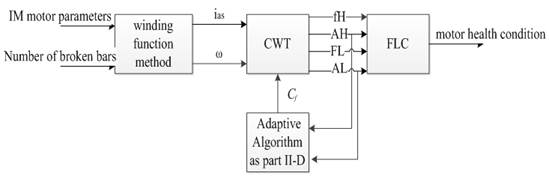Figure 1. Overall control algorithm to train the FLC in simulation.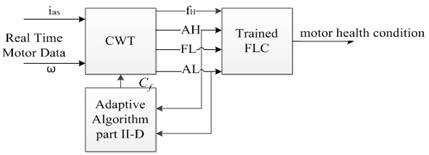Figure 2. Overall control scheme to detect the motor fault.

B.  Continuous Wavelet Transform (CWT)

Continuous wavelet coefficients of an arbitrary function x(t) are calculated from the integration of the basic wavelet function between (-∞,+∞). According to the definition, wavelet coefficients are calculated as below.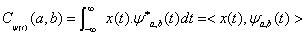(1)

where, Ψa,b is the basic functions of the wavelet transform that is obtained from the main wavelet: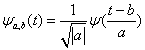(2)

where, "a" and "b" are the scale and displacement factors respectively. Ψ (t) is the Wavelet function which can be calculated from the following conditional equations:(3)

where,is the Fourier transform of.

Application of the Gaussian continuous wavelet function increases the accuracy of harmonic calculation significantly. The mathematical function of main Gaussian wavelet is written as follows: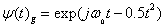(4)

where,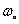is the fundamental frequency of Gaussian wavelet function.

C.  Frequency Calculation of Harmonics

Substituting the Gaussian wavelet function equation (4) in equation (1), yield,(5)

where,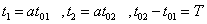In the above equation, T is wavelet function time. Considering (5), the harmonics frequency is obtained as,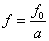(6)

where, f0 in the fundamental frequency of the wavelet function. In this paper, the scale factor "a" will be maximized at the lowest detected frequency (fL) and it will be minimized at the highest detected frequency (fH). Thus, the following equation is obtained: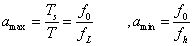(7)

where, Ts is the signal processing time. Using (6), (7) scale factor can be obtained as,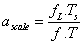(8)

where,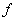is the frequency, which is supposed to be detected.

D. Amplitude and Phase Calculation of Harmonics

Correction factors have a critical role in the accuracy of harmonic amplitude calculation. The amount and number of these coefficients depend on the detected bandwidth frequency. In fact, the accuracy of the harmonic calculation by using CWT depends on the estimated coefficients for the desired frequency bandwidth.

Considering (5), amplitude and phase of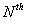harmonic are obtained as following equation.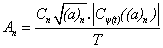(9)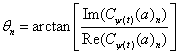(10)

where, "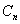" is correction factors ofharmonic.

To calculate the correction factors in a specified frequency bandwidth, a sinusoidal single-frequency signal with desired frequency of the correction factors with unity amplitude is considered as the input signal. The largest values of the calculated wavelet coefficients show the powerful corresponding frequency in the signal. Since the input is a single frequency signal with unity amplitude, replacing one as the value of the harmonic amplitude in (9) leads to the desired frequency correction factor. So, the correction factor for a frequencyis obtained as below.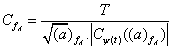(11)

The linear interpolation method is then used to calculate correction factors in the bandwidth frequency and for some frequencies in the form of integer or rational numbers in between two frequencies, depending on the desired accuracy. The obtained factors are used to correct the fault frequency amplitudes in different broken bar fault and load conditions.

Correction factors depend on the resolution of scale factor, displacement factor, minimum and maximum detected frequencies and sampling frequency. These parameters are calculated by using trial and error method. The dependency of the correction factors on those parameters can be eliminated by selecting the appropriate frequency bandwidth to detect the fault precisely. However, the sensitivity of correction factors is different for each parameter.

F.  Calculation of Fault Frequencies

The frequency components of the fault can be expressed as,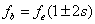(12)

Where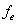main frequency and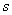is slip coefficient. Considering the stator current spectrum which obtained from the proposed adaptive CWT, the frequencies and amplitudes corresponding to different broken bar fault conditions are calculated accurately using the following steps:

step1: Calculate the fault frequencies from the equation (12) (fb).

step2: Calculate the maximum and the minimum speed in steady state to obtain the frequency variation domain Δf = maximum frequency – minimum frequency.

Step3: Search for the maximum frequency amplitude in interval (fb- Δf, fb+ Δf) for upper and lower side fault frequencies from the stator current spectrum.

Step5: Search the corresponding frequencies to maximum amplitudes of the stator current spectrum.

Step6: Save the amplitudes and frequencies of the last 2steps.

Step7: If the possibilities for all different broken bar fault and load conditions are considered go to stop, if not go to Step1.

In the above steps, the slip frequency is calculated accurately in different broken bar fault and load conditions which concluded the appropriate accuracy of fault detection frequency.

3. Design of Specific Fuzzy Logic Controller

As shown in Figure 1, the inputs to the FLC are low side frequencies (fL), high-side frequencies (fh) and their amplitude (AL), (Ah) and the output is the number of broken rotor bars. The structure of the FLC is shown in Figure 3. The output of the FLC can be expressed mathematically as a function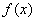as follows:(13)

where,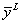and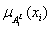are initializing value and degree of each membership function,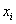respectively.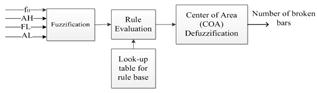Figure 3. The FLC structure.

The membership functions of inputs include fL, fh and their amplitude (AL), (Ah), is shown in Figure 4. Interval of these membership functions and their fuzzy sets are shown in (14)-(17), respectively.(14)(15)(16)(17)

where, the "min" and "max" values are determined from all the values obtained from CWT analysis. Output membership functions are selected by trial and error as shown in Figure 5. Also, output of fuzzy system which is the number of broken bars varies from 0 to 10 and is defined as below.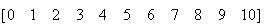(18)

Fuzzy rules are generally in the form of if-then which ith rule is written as below.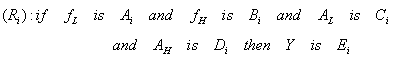(19)

TABLE 1 Lists fuzzy rules based look-up table resulted from CWT analysis. There are total 48 rules which are generated based on simulation tests with known data.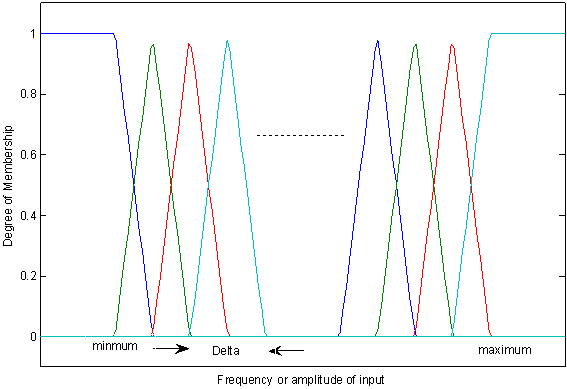Figure 4. Input membership function for the FLC.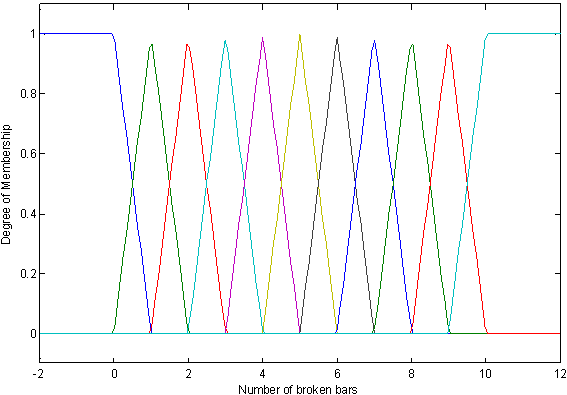Figure 5. Output membership function for the FLC.

4. Training Algorithm for FLC

Fuzzy logic controller (FLC) is trained based on the output of the adaptive CWT as shown in Figure 2. In the training process the triangular membership functions is changed to Gaussian functions to obtain the optimum output based on gradient steepest descent training method.

The objective of training the FLC membership functions is to determine the functionso that the error between desired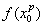and actual output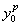is minimized.(20)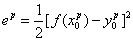(21)

In (20),,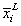, and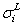are initializing value and in (21)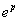is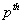output error. To achieve this goal these steps are needed:

Step1: Identifying fuzzy rules and initializing values,, and.

Step2: Outputs calculation based on the inputs of fuzzy model in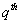input step as shown in (22)-(24).

Table 1. Rule based matrix for the flc.

 Rule Number Ai Bi Ci Di Ei 1 100 62 2 1 1 2 113 52 5 4 2 3 107 59 19 11 3 4 104 53 10 6 3 5 107 54 1 3 3 6 107 53 4 4 3 7 104 52 10 8 3 8 103 52 17 6 3 9 107 59 11 5 3 10 107 58 7 2 3 11 107 54 3 2 3 12 107 54 6 3 3 13 106 52 13 4 3 14 103 52 19 6 3 15 109 59 14 7 3 16 109 58 12 1 3 17 91 76 24 19 4 18 97 68 16 17 4 19 97 67 14 22 4 20 101 52 19 14 4 21 106 60 10 11 4 22 97 67 8 19 4 23 100 68 20 10 4 24 106 61 7 11 4 25 73 87 64 39 5 26 88 76 4 9 5 27 88 61 15 9 5 28 91 76 22 22 5 29 88 76 8 8 5 30 94 67 2 3 5 31 91 76 17 18 5 32 91 62 37 29 5 33 85 73 18 18 5 34 90 67 22 23 5 35 85 74 35 25 5 36 81 73 9 17 5 37 91 68 37 31 5 38 44 117 114 1 2 39 68 83 49 23 2 40 69 99 69 36 2 41 72 90 69 50 2 42 72 91 55 41 2 43 69 99 74 37 2 44 84 83 7 12 2 45 79 74 28 26 2 46 79 90 56 29 2 47 78 91 26 9 2 48 78 74 57 17 2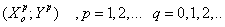(22)(23)(24)

Step3: Parameters correction instep (identifying parameters value in step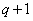) as shown in (25-29)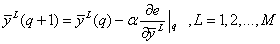(25)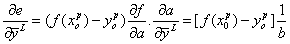(26)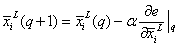(27)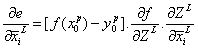(28)(29)

Step4: Returning to second step and replacing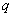withto decrease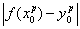value to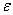or reaching to determined error.

Step5: Returning to second step and replacing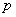with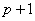to calculate parameters of new inputs for input-output pair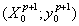.

Step6: Replacingwith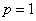and repeating steps 4 and 5 to obtain the better fuzzy model output.

5. Simulation Results

First, a sample comparison of the proposed adaptive CWT and FLC based analysis with the conventional FFT based analysis is done based on distorted signal with main frequency equal to 50Hz. Amplitudes and phases of harmonic frequencies were chosen arbitrarily. The comparative results are shown in TABLE 2. From TABLE 2, it is found that the amplitude, frequency, and especially phase accuracy of the harmonics in the proposed method is more accurate than FFT method .

Table 2. Comparison of the proposed CWT and conventional FFT methods.

 Frequency (Hz) Amplitude (A) Phase (degree) true values FFT The adaptive CWT true values FFT The adaptive CWT true values FFT The adaptive CWT 25 24.99 25 0.3 0.3 0.3 70 81.45 70.0 35.35 35.3 35.36 0.2 0.17 0.2 60 137.8 60.29 35.85 35.82 35.85 0.7 0.62 0.7 80 124.53 79.98 50 49.99 50.01 1.0 0.97 1.0 0 22.39 0.01 86.6 86.64 86.62 0.5 0.38 0.5 90 18.09 89.99 150 149.96 150.02 0.4 0.31 0.40 40 107.37 40.00

The performance of the proposed CWT and FLC based fault detection method is tested in simulation at different load and broken bar fault conditions. The motor parameters used for simulation is shown in TABLE 3.

Table 3. Test motor specifiactions.

 Motor Specifications Parameters Value Parameters Value PR 4 kW VL-L 400 V f 50 Hz Pole Paris 2 NR 1380 rpm Rs 1.4 Ω Ls 0.0058 Ω RR 1.4 Ω LR 0.0058 Ω Lm 0.17 Ω J 0.05 Kg.m2 F 0.004 Kg.m2/s

The motor with more than five broken bars is not required to be discussed, because in this case the motor fault and mal-operation is obvious. That is why; various simulated conditions only include healthy status, one broken bar, two broken bars with 17 permutation, three broken bars with 8 permutation, four broken bars with 13 permutation and five broken bars with 11 permutation, which in total of 51 permutation. Some sample results are presented below.

Figure 6 shows simulation of stator current and motor speed for both healthy and faulty motor with two broken bars. The winding function theory is used to calculate the stator current and speed. It is hard to distinguish between healthy and faulty motors when there are only two broken rotor bars.

Figure 7 illustrates the frequency spectrum of the stator current for healthy and faulty motor with five broken bars at full load while stator current for each condition with 600 Hz

sampling frequency and selective bandwidth frequency from 20 to 300 Hz is processed with CWT and frequency spectrum is obtained in each condition using trial and error method.

As seen in Figure 7 (a), the frequency components around the main frequency cannot be seen apparently. But, the frequencies with the very low amplitudes can be found around the main frequency according to the mentioned steps in section ІІ-F. From Figure 7 (b), the low and high side frequencies can be clearly seen around the main frequency which obtained from the mentioned steps in section ІІ-F. From Figure 7 (b), it can also be seen that the rated slip frequency is increased due to faulty motor with five broken bars based on equation 12 (s=13%) as compared to the healthy motor based on TABLE 3 (s=8%).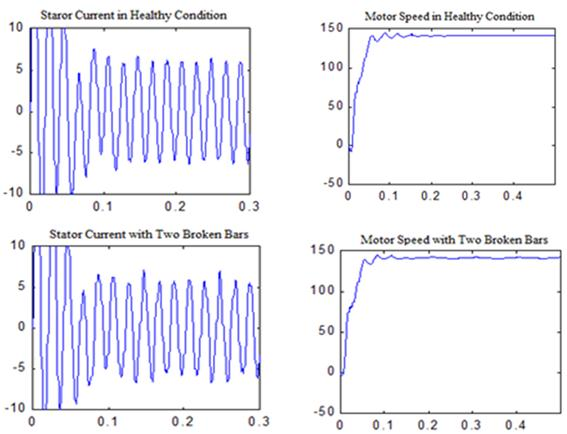Figure 6. Motor speed and current responses for both healthy and faulty motors with two broken bars based on modified WFM method.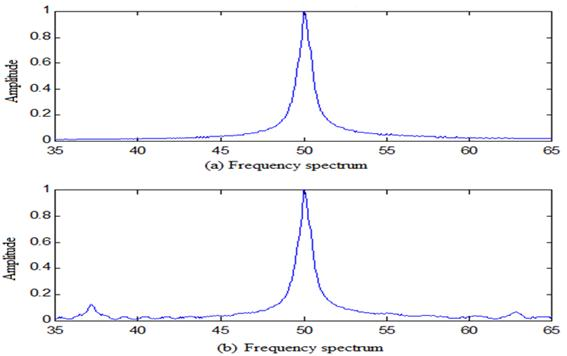Figure 7. Stator current spectrum for healthy (a) and faulty motor with five broken bars (b) at full load for the proposed adaptive CWT method.

Figure 8 compares the harmonic amplitudes at low and high frequencies from healthy condition to five broken bars of the simulated motor for the proposed adaptive CWT. Figure 9 and Figure 10 show comparison between FFT and the proposed adaptive CWT for low side amplitudes and their frequency, respectively from healthy condition to five broken bars. It is seen clearly from Figs. 8, 9 that the harmonic amplitudes are increasing with the increased number of rotor broken bars. It is also seen from Figure 8 that the low side amplitudes are always higher than the high side amplitudes due to the currents asymmetry, which is risen by increasing the number broken bars . From Figure 9, it is also seen that the harmonic amplitudes obtained from both FFT and the proposed adaptive CWT analysis are not same due to the inaccuracy in FFT, which was shown earlier in TABLE 2.

With increasing the number of the rotor broken bars, the produced torque by the rotor bars is decreased. Therefore, the motor speed is reduced which decreases the low side frequencies as shown in Figure 10 for both FFT and the proposed adaptive CWT methods. However in the healthy motor, unlike to the faulty motor, the speed variations are almost equal to zero and the value of the low side frequency does not follow these variations which can be clearly seen in Figure 10 for the proposed method. Furthermore it is clearly found out from Figure 10, the differences of the low side frequencies between the FFT and the proposed adaptive CWT methods are increasing with the increased number of the rotor broken bars. These differences are related to the speed variations which considered for calculating of the harmonic components in the proposed method. It is noteworthy that with increasing the number of the rotor broken bars, the speed variations are increased. Under the foretold scenario, the proposed method shows a high accuracy to calculate the harmonic components and it is more accurate than the conventional FFT.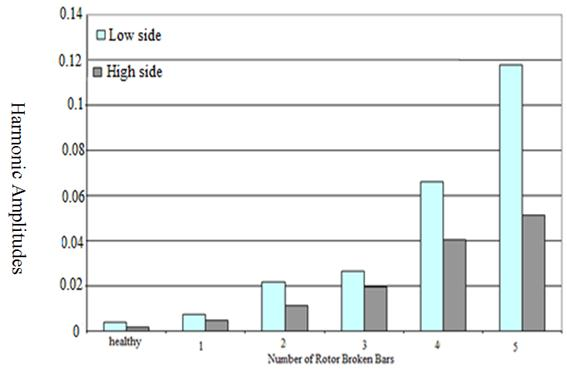Figure 8. Comparison between high and low side amplitudes of the proposed adaptive CWT from healthy to five broken bars.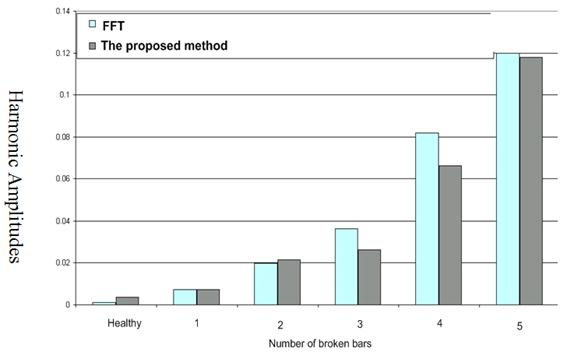Figure 9. Comparison of the low side amplitudes between FFT and the proposed adaptive CWT method from healthy condition to five broken bars.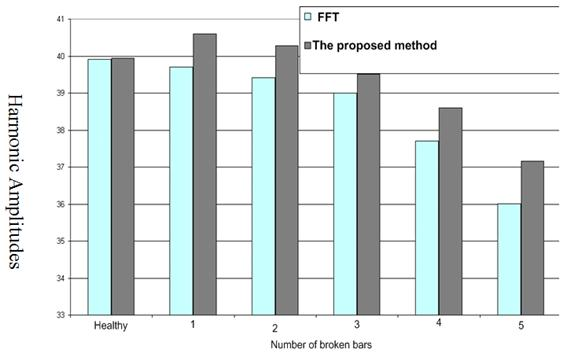Figure 10. Comparison of the low side frequencies between FFT and the adaptive CWT proposed method from healthy condition to five broken bars.

To show the performance of the trained FLC, the tested data including harmonic components of 3 broken bars (1, 6, 7), 4 broken bars (1, 8, 9, 10), and 5 broken bars (1, 11, 12, 13, 14) at full load is used as inputs for the FLC. TABLE 4 shows Output results of the trained FLC for simulation tests of the proposed method. This Table confirm the proper performance of the trained FLC and adaptive CWT. It is found that the proposed trained FLC can detect the fault accurately in simulation. Traditionally in FFT, the fault frequencies are calculated based on (12) and the constant slip, which is calculated based on average steady-state speed of the motor. However, the slip changes during fault condition as the motor speed changes. Therefore, the conventional FFT method provides inaccurate result for fault frequencies. In this paper, the adaptive CWT method is used to calculate the accurate fault frequency based on the Steps mentioned in section ІІ-F. Finally, after finding the frequency range and side frequencies through slip calculation, actual high and low frequencies and the corresponding stator current amplitudes are obtained.

6. Experimental Result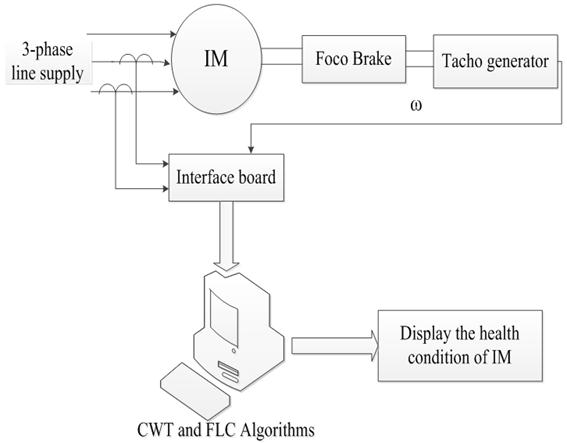Figure 11. Hardware schematic for experimental tests of the proposed fault detection algorithm.

Figure 12 and Figure 13 illustrate the experimental frequency spectrum of the stator current for healthy and faulty motor with five broken bars at no load and very high load, respectively. It is clearly seen that there is no harmonic content for healthy condition. But for faulty motor there are low and high side harmonic contents in stator current.

Figure 14 shows low and high side frequency amplitudes of the proposed method from healthy condition to five broken bars in experimental test. It is found from Figure 14 that the low side amplitudes are higher than high side amplitudes in real time, which also validates the simulation results.

Figure 15 shows low frequency amplitudes between simulation and experimental results from healthy condition to five broken bars for the proposed method. It is found from Figure 14 that the harmonic amplitudes in real-time is higher than simulation results. It is due to the welding of broken bars in several tests which increases the resistance of rotor bars more than its original value.

The motor is tested for healthy, 1, 3, and 5 broken rotor bars at different load conditions. Table 5 shows the summary of experimental test to diagnose the healthy or faulty condition of the motor at different load conditions. It is found from Table 5 that the proposed adaptive CWT and trained FLC can detect the healthy condition or number of broken rotor bars for IM accurately in real-time.

Table 4. Output result of the trained FLC for simulation test of the IM with broken rotor bars.

 The trained FLC input The trained FLC diagnose Upper side amplitude Upper side frequency Lower side amplitude Lower side frequency Broken bar 3 broken bars 0.0098 60.3743 0.0205 39.9388 1,6,7 4 broken bars 0.0271 60.3197 0.0359 39.5127 1,8,9,10 5 broken bars 0.0421 61.4880 0.0654 38.5307 1,11,12,13,14Figure 12. Stator current spectrum in healthy condition in no load for the experimental test of proposed method.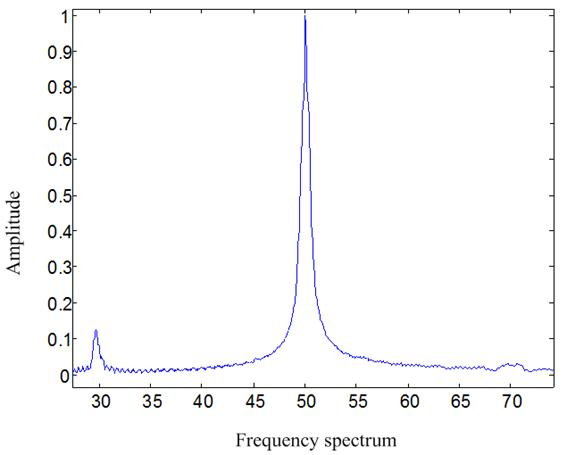Figure 13. Stator current spectrum with five broken bars in very high load for the experimental test of proposed method.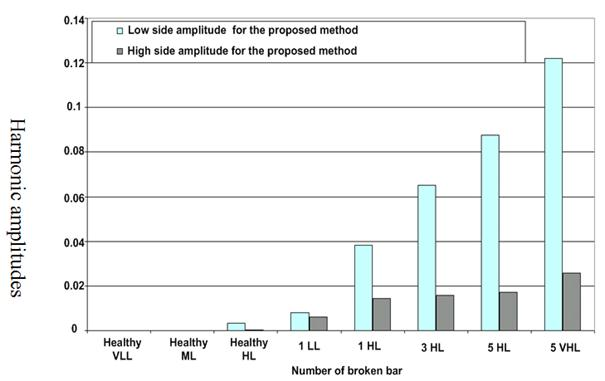Figure 14. Low and high side frequency amplitudes of the proposed model from healthy condition to five broken bars in experimental test.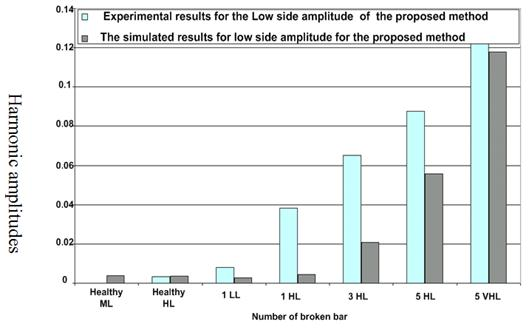Figure 15. Comparison of low side amplitudes between simulation and experimental results from healthy condition to five broken bars for the proposed method.

Table 5. Output result of the trained FLC for analysed motor in experimental test.

 Input to the FLC Fuzzy system diagnose Upper side amplitude Upper side frequency Lower side amplitude Lower side frequency Load condition Healthy motor 0 50.3398 0 49.6648 Very low load Healthy motor 0 55.1268 0.0001 44.8531 Medium load Healthy motor 0.0002 60.6428 0.0032 39.2773 High load 1 broken bar 0.0061 52.6870 0.0081 47.3037 Very low load 1 broken bar 0.0144 62.9921 0.0383 36.7447 High load 3 broken bars 0.0158 62.7549 0.0652 32.8030 High load 5 broken bars 0.0172 63.1712 0.0875 36.6435 High load 5 broken bars 0.0259 70.6714 0.1219 29.6780 Very high load

7. Conclusion

An online adaptive continuous wavelet transform and fuzzy logic based high precision fault detection method for three phases IM has been presented in this work. Based on the motor current signature analysis at different broken bar fault and load conditions, the CWT generates the harmonic amplitudes and frequencies of the motor stator current. The coefficients of CWT are adapted online based on the harmonics amplitude, which are the output of CWT. Based on this information, first the FLC is trained in simulation. Then, the trained FLC detects faulty or healthy condition of the IM in real time. The results found that the proposed adaptive CWT based analysis is more accurate than the conventional FFT based analysis to detect the harmonic components of a signal and the proposed adaptive CWT and FLC based fault detection method can detect the IM fault accurately. Therefore, this method could be a potential candidate for real-time fault detection.

References

1. S. S. Sebtahmadi, H. Pirasteh, S. H. Aghay Kaboli, A. Radan and S. Mekhilef, "A 12-Sector Space Vector Switching Scheme for Performance Improvement of Matrix-Converter-Based DTC of IM Drive," in IEEE Transactions on Power Electronics, vol. 30, no. 7, pp. 3804-3817, July 2015.
2. M. Riera-Guasp, M. F. Cabanas, J. A. Antonino-Daviu, M. Pineda-Sanchez, and C. Garcia, "Influence of Nonconsecutive Bar Breakages in Motor Current Signature Analysis for the Diagnosis of Rotor Faults in Induction Motors," Energy Conversion, IEEE Transactions on, vol. 25, pp. 80-89, 2010.
3. K. S. Gaeid, H. W. Ping, M. K. Masood, and M. A. Saghafinia, "Induction motor fault tolerant control with wavelet indicator," in Transportation, Mechanical, and Electrical Engineering (TMEE), 2011 International Conference on, 2011, pp. 949-953.
4. P. Konar and P. Chattopadhyay, "Bearing Fault Detection of Induction Motor using Wavelet and Support Vector Machines (SVM)," Applied Soft Computing, 2011.
5. A. Saghafinia, H. W. Ping, and M. Rahman, "High performance induction motor drive using hybrid fuzzy-pi and pi controllers: A review," International Review of Electrical Engineering-Iree, vol. 5, pp. 2000-2012, 2010.
6. S. H. Kia, H. Henao, and G. A. Capolino, "Diagnosis of broken-bar fault in induction machines using discrete wavelet transform without slip estimation," Industry Applications, IEEE Transactions on, vol. 45, pp. 1395-1404, 2009.
7. J. Cusido, L. Romeral, J. A. Ortega, J. A. Rosero, and A. Garcia Espinosa, "Fault detection in induction machines using power spectral density in wavelet decomposition," Industrial Electronics, IEEE Transactions on, vol. 55, pp. 633-643, 2008.
8. J. Zhang, P. Shi, and Y. Xia, "Robust adaptive sliding-mode control for fuzzy systems with mismatched uncertainties," Fuzzy Systems, IEEE Transactions on, vol. 18, pp. 700-711, 2010.
9. A. Saghafina, H. W. Ping, M. N. Uddin, and K. S. Gaied, "Adaptive fuzzy sliding-mode control into chattering-free induction motor drive," in Industry Applications Society Annual Meeting (IAS), 2012 IEEE, 2012, pp. 1-8.
10. A. Saghafinia, H. Ping, and M. Uddin, "Designing Self-Tuning Mechanism On Hybrid Fuzzy Controller For High Performance And Robust Induction Motor Drive," the International Journal of Advanced Technology & Engineering Research, vol. 3, 2013.
11. A. Saghafinia and H. W. Ping, "High performance induction motor drive using fuzzy self-tuning hybrid fuzzy controller," in Power and Energy (PECon), 2010 IEEE International Conference on, 2010, pp. 468-473.
12. A. Saghafinia, H. W. Ping, and M. N. Uddin, "Sensored field oriented control of a robust induction motor drive using a novel boundary layer fuzzy controller," Sensors, vol. 13, pp. 17025-17056, 2013.
13. A. Saghafinia, H. W. Ping, and M. N. Uddin, "Fuzzy sliding mode control based on boundary layer theory for chattering-free and robust induction motor drive," The International Journal of Advanced Manufacturing Technology, vol. 71, pp. 57-68, 2014.
14. A. Saghafinia, H. W. Ping, M. N. Uddin, and K. S. Gaeid, "Adaptive Fuzzy Sliding-Mode Control Into Chattering-Free IM Drive," Industry Applications, IEEE Transactions on, vol. 51, pp. 692-701, 2015.
15. Z. H. Salih, K. S. Gaeid, and A. Saghafinia, "Sliding Mode Control of Induction Motor with Vector Control in Field Weakening," Modern Applied Science, vol. 9, p. p276, 2015.
16. Kaboli, S. Hr A., et al. "A hybrid adaptive Neural-Fuzzy tuned PI controller based Unidirectional Boost PFC converter feeds BLDC drive." Power Electronics, Drive Systems and Technologies Conference (PEDSTC), 2013 4th. IEEE, 2013.
17. Mansouri, Mahdi, et al. "A hybrid Neuro-Fuzzy—PI speed controller for BLDC enriched with an integral steady state error eliminator." Control System, Computing and Engineering (ICCSCE), 2012 IEEE International Conference on. IEEE, 2012.
18. A. M. Takbash, J. Faiz, and B. M. Ebrahimi, "Losses Characterization in Voltage-Fed PWM Inverter Induction Motor Drives Under Rotor Broken Bars Fault," Magnetics, IEEE Transactions on, vol. 49, pp. 1516-1525, 2013.
19. S. Nandi, S. Ahmed, and H. A. Toliyat, "Detection of rotor slot and other eccentricity related harmonics in a three phase induction motor with different rotor cages," Energy Conversion, IEEE Transactions on, vol. 16, pp. 253-260, 2001.
20. W. Xu, G. Sun, G. Wen, Z. Wu, and P. K. Chu, "Equivalent Circuit Derivation and Performance Analysis of a Single-Sided Linear Induction Motor Based on the Winding Function Theory," Vehicular Technology, IEEE Transactions on, vol. 61, pp. 1515-1525, 2012.
21. A. Saghafinia, "The Use of Wavelet Transform and Fuzzy Predictor for Rotor Fault Detection in Three Phase Induction," Master, Department of Electrical and Computer Engineering, Isfahan University of Technology, Isfahan, Iran, 2001.

 Contents 1. 2. 3. 4. 5. 6. 7.
Article ToolsAbstractPDF(751K)